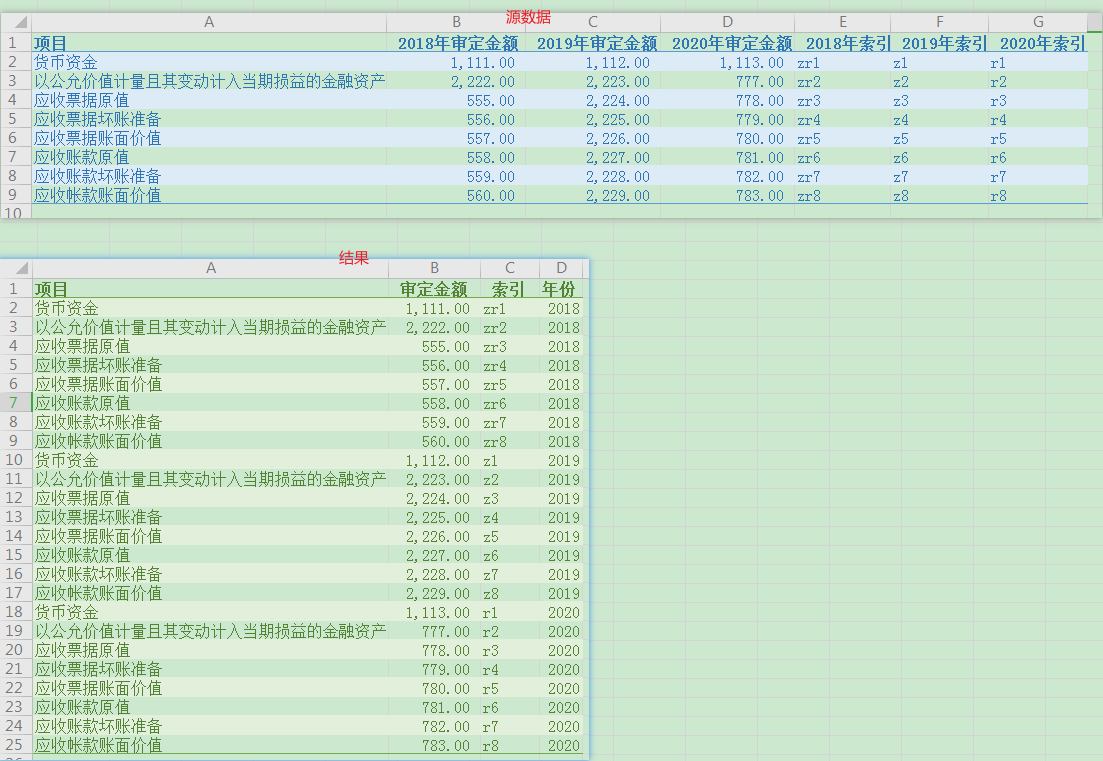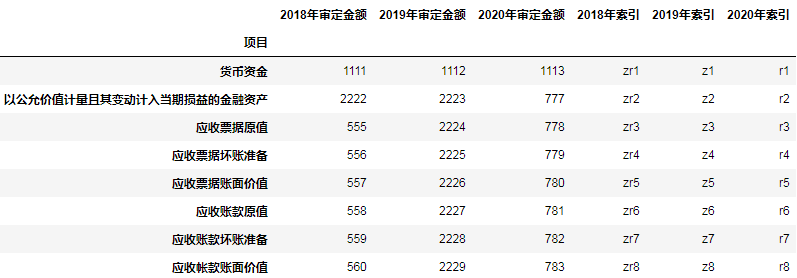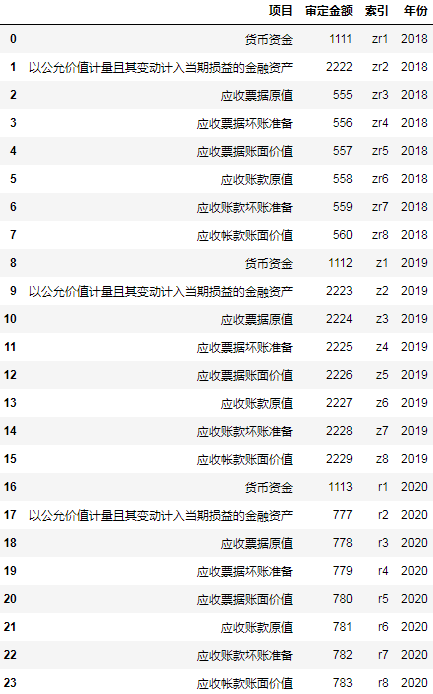``````import pandas as pd

df
``````groupby分组相信大部分读者都使用过，但一直都是按行分组，不过groupby不仅可以按行分组，还可以按列进行分组。

``````result = []
for year, split in df.groupby(df.columns.str[:4], axis=1):
split.rename(columns=lambda s: s[5:], inplace=True)
split.reset_index(inplace=True)
split["年份"] = year
result.append(split)
result = pd.concat(result, ignore_index=True)
result
``````Pandas指定样式保存excel数据的N种姿势

``````df.columns.str[:4]
``````

``````Index(['2018', '2019', '2020', '2018', '2019', '2020'], dtype='object')
``````

``````split.rename(columns=lambda s: s[5:], inplace=True)
``````

``````split.reset_index(inplace=True)
``````

``````split["年份"] = year
``````